# Scientific Notation section 5 6 Scientific Notation is

• Slides: 14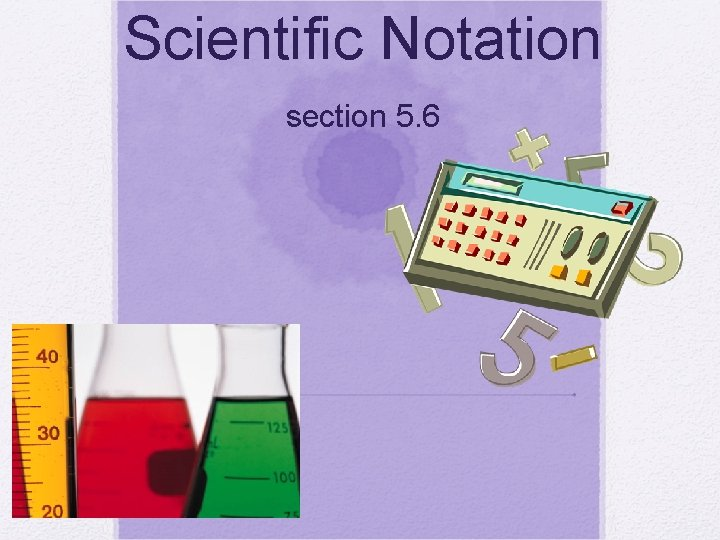Scientific Notation section 5. 6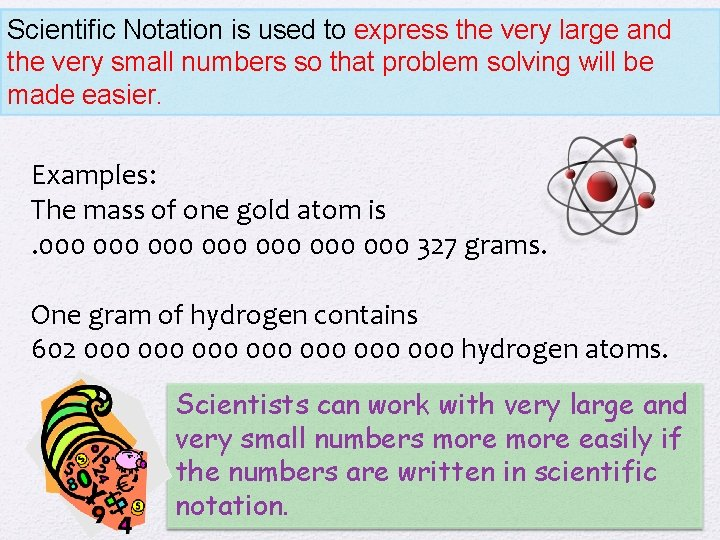Scientific Notation is used to express the very large and the very small numbers so that problem solving will be made easier. Examples: The mass of one gold atom is. 000 000 327 grams. One gram of hydrogen contains 602 000 000 hydrogen atoms. Scientists can work with very large and very small numbers more easily if the numbers are written in scientific notation.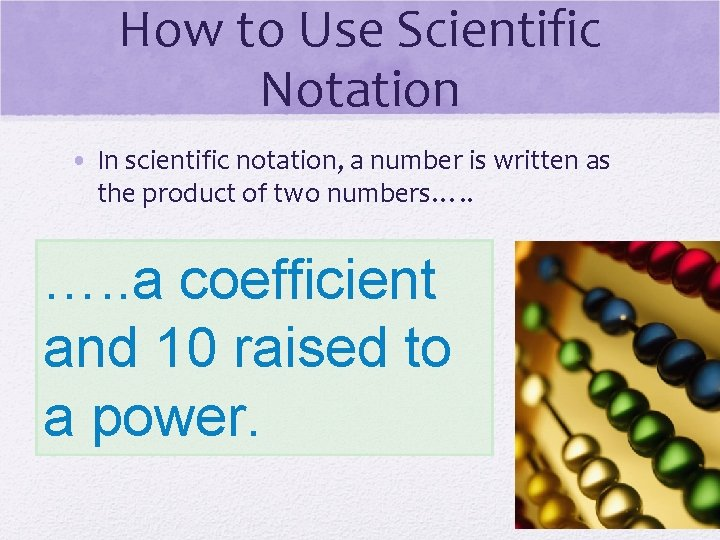How to Use Scientific Notation • In scientific notation, a number is written as the product of two numbers…. . a coefficient and 10 raised to a power.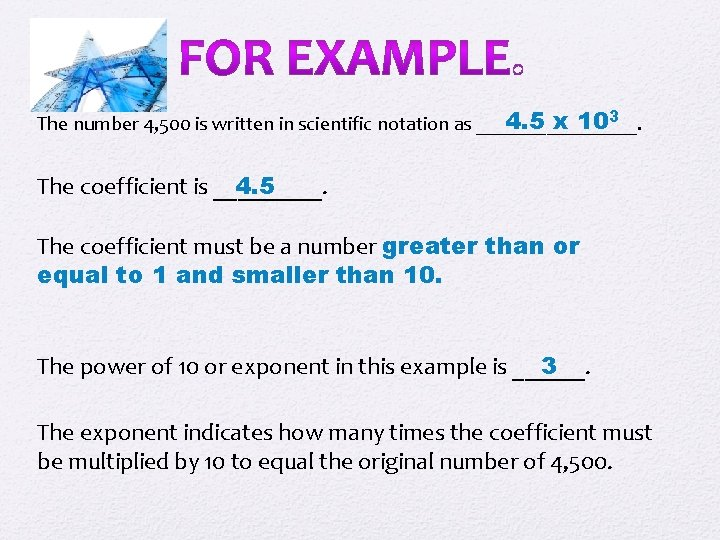4. 5 x 103 The number 4, 500 is written in scientific notation as ________. The coefficient is _____. 4. 5 The coefficient must be a number greater than or equal to 1 and smaller than 10. The power of 10 or exponent in this example is ______. 3 The exponent indicates how many times the coefficient must be multiplied by 10 to equal the original number of 4, 500.If a number is greater than 10, the exponent will be positive _______ and is equal to the number of places the decimal must be moved to left the ____ to write the number in scientific notation.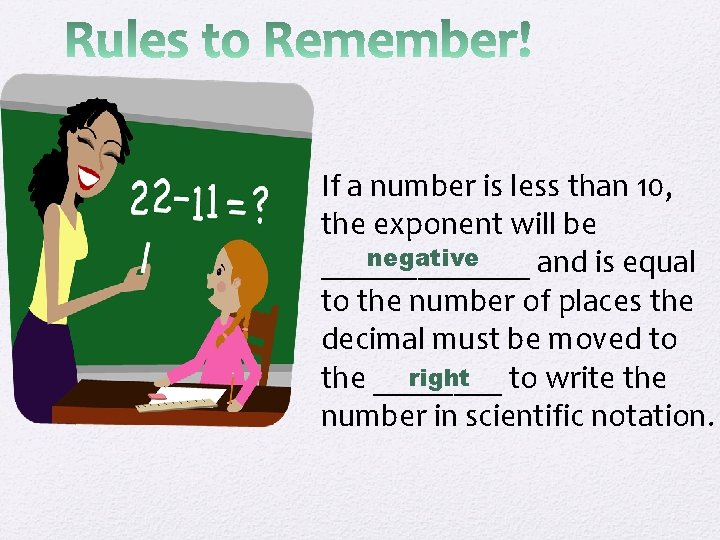If a number is less than 10, the exponent will be negative _______ and is equal to the number of places the decimal must be moved to right the ____ to write the number in scientific notation.A number will have an exponent of zero if: …. the number is equal to or greater than 1, but less than 10.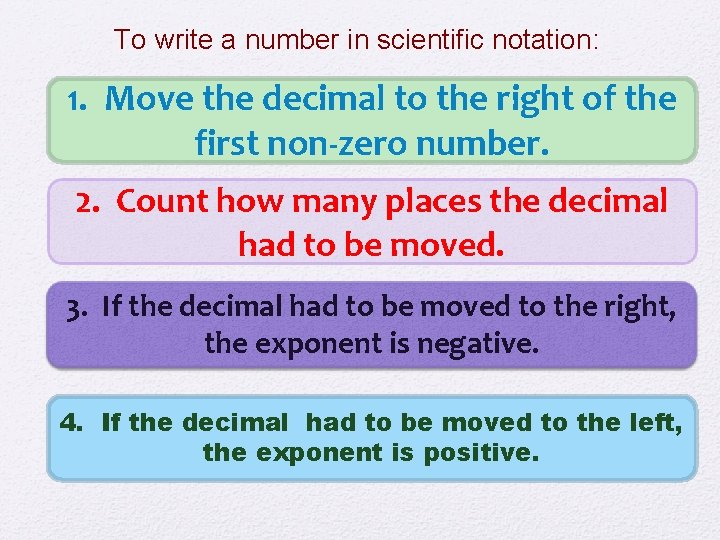To write a number in scientific notation: 1. Move the decimal to the right of the first non-zero number. 2. Count how many places the decimal had to be moved. 3. If the decimal had to be moved to the right, the exponent is negative. 4. If the decimal had to be moved to the left, the exponent is positive.Practice Problems Put these numbers in scientific notation. PROBLEMS 1). 00012 2) 1000 3) 0. 01 4) 12 5). 987 6) 596 7). 000 7 8) 1, 000 9). 001257 10) 987, 653, 000 11) 8 ANSWERS 1) 1. 2 x 10 -4 2) 1 x 103 3) 1 x 10 -2 4) 1. 2 x 101 5) 9. 87 x 10 -1 6) 5. 96 x 102 7) 7. 0 x 10 -7 8) 1. 0 x 106 9) 1. 26 x 10 -3 10) 9. 88 x 1011 11) 8 x 100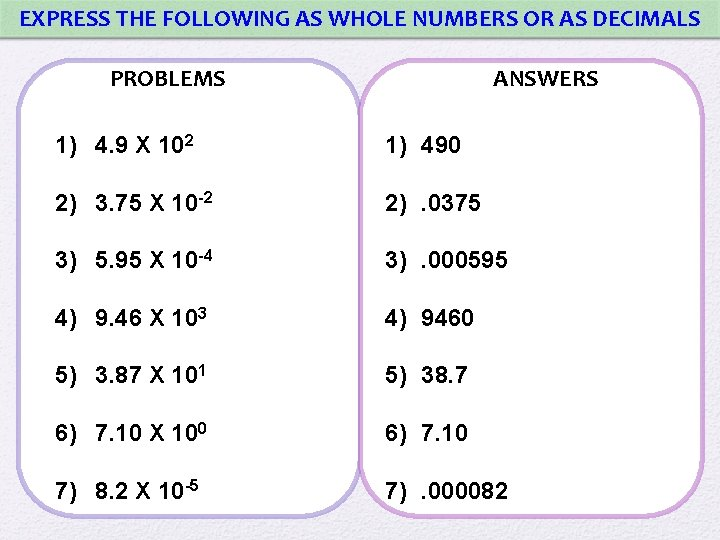EXPRESS THE FOLLOWING AS WHOLE NUMBERS OR AS DECIMALS PROBLEMS ANSWERS 1) 4. 9 X 102 1) 490 2) 3. 75 X 10 -2 2). 0375 3) 5. 95 X 10 -4 3). 000595 4) 9. 46 X 103 4) 9460 5) 3. 87 X 101 5) 38. 7 6) 7. 10 X 100 6) 7. 10 7) 8. 2 X 10 -5 7). 000082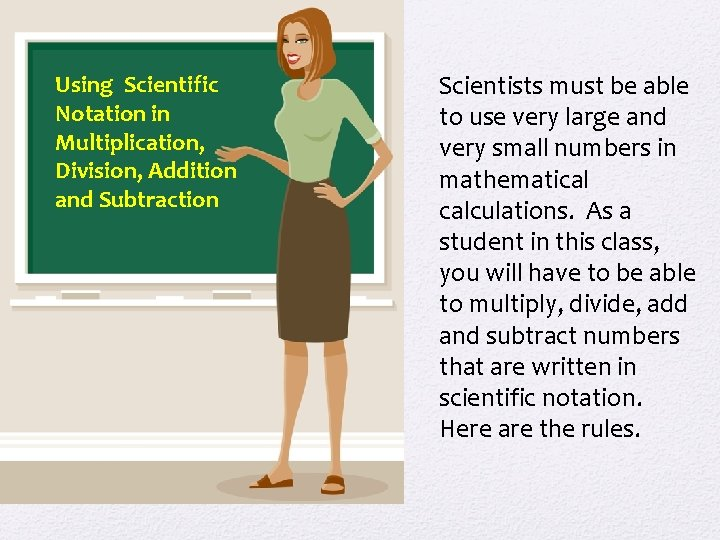Using Scientific Notation in Multiplication, Division, Addition and Subtraction Scientists must be able to use very large and very small numbers in mathematical calculations. As a student in this class, you will have to be able to multiply, divide, add and subtract numbers that are written in scientific notation. Here are the rules.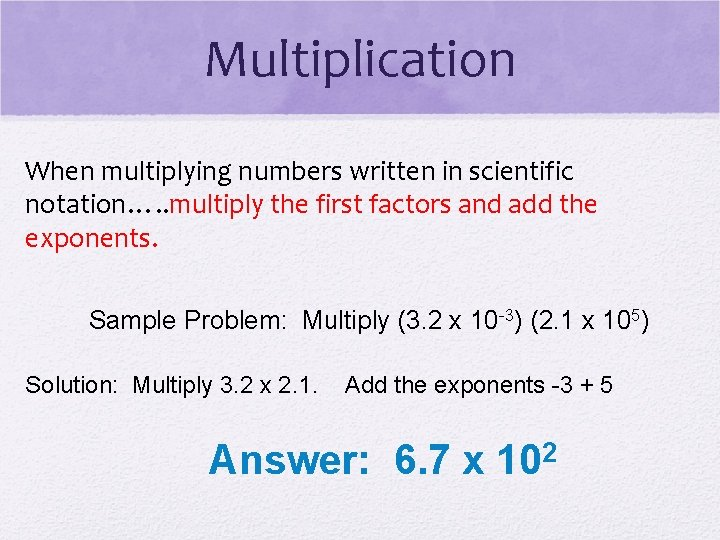Multiplication When multiplying numbers written in scientific notation…. . multiply the first factors and add the exponents. Sample Problem: Multiply (3. 2 x 10 -3) (2. 1 x 105) Solution: Multiply 3. 2 x 2. 1. Add the exponents -3 + 5 Answer: 6. 7 x 102Division Divide the numerator by the denominator. Subtract the exponent in the denominator from the exponent in the numerator. Sample Problem: Divide (6. 4 x 106) by (1. 7 x 102) Solution: Divide 6. 4 by 1. 7. Subtract the exponents 6 - 2 Answer: 3. 8 x 104Addition and Subtraction To add or subtract numbers written in scientific notation, you must…. express them with the same power of ten. Sample Problem: Add (5. 8 x 103) and (2. 16 x 104) Solution: Since the two numbers are not expressed as the same power of ten, one of the numbers will have to be rewritten in the same power of ten as the other. 5. 8 x 103 =. 58 x 104 so. 58 x 104 + 2. 16 x 104 =? Answer: 2. 74 x 104